# How to Compare Two Columns in Excel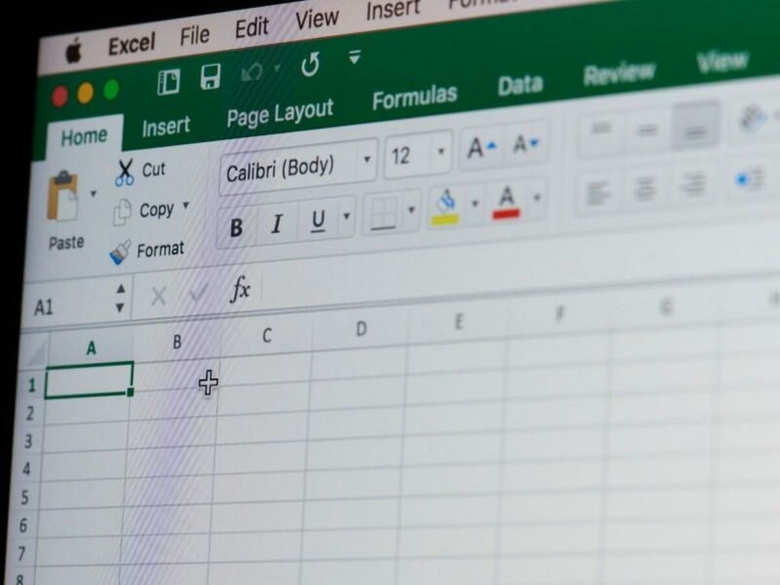Spreadsheets mainly comprise of tabulated data, so it is only natural that at some point a comparison between the data contained in two columns is going to be required.

The data may be weekly or monthly sales figures in a report and there is a need to compare the current period’s figures against those of the previous to produce some kind of indicator.

Have sales gone up, have they gone down, or have they remained the same?

As with any good software, Excel provides a few ways to perform comparisons and we’ll be taking a close look at these.

Before we dive in though, it would be a good idea to first get a basic understanding of Excel’s comparison operators.

## Excel Comparison Operators

Apart from comparing two values to determine whether they are the same or not, comparisons can also be made to see whether one value is greater or less than another value, as well as whether a value is greater than or equal to, and less than or equal to another value.

There are six operators available in Excel, that allow a comparison between the values contained in two cells.

They are listed in the table below.

Comparisons result in a TRUE or FALSE being returned (referred to as a boolean type), although as we’ll see later, the output can also be programmed to produce something more meaningful.

Comparison operator Meaning Example

=

Equal to =A1=B1

>

Greater than =A1>B1

<

Less than =A1<B1

>=

Greater than or equal to =A1>=B1

<=

Less than or equal to =A1<=B1

<>

Not equal to =A1<>B1

## Basic Comparisons

The following is a sample sheet with two columns containing cells with random numbers (between 10 and 19 inclusive), as well as some cells with text.

We will be using this sheet as our sample data to show how the comparison operators work.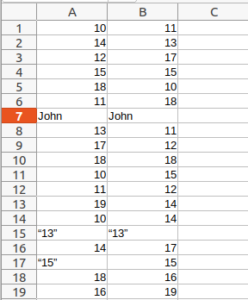Inspecting the sheet visually looking for equal values, we can see that columns A and B have a match at rows 4, 7, 10, and 15.

While doing a visual comparison may work for a small range of data, it is far more common for spreadsheets to comprise large sets of data spanning a hundred or more rows.

While looking for values that are equal (or not) is relatively easy, looking for whether the value in one cell is less than, more than, less than or equal to, more than or equal to the value in another cell, creates an even greater degree of difficulty.

And if that’s not enough, the columns containing the data may not necessarily be next to each other, making visual comparison extremely difficult, slow, and more importantly, error prone.

So let’s leverage the real power of spreadsheets, that of formulas.

Referring to the comparison operators table, in the example column when comparing for equality between two cells, the formula is given as follows:

=A1=B1

The first equals sign merely indicates that this is a formula, while the formula itself is testing for whether the contents of cell A1 are equal to the contents of cell B1.

In our sample sheet, cell A1 contains the number 10 and cell B1 contains the number 11.

Hence, they are not equal.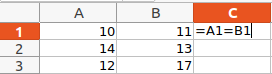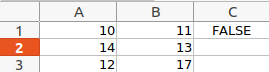To display the results of the comparison of adjacent cells between column A and column B, we will use column C.

We can thus start by entering our formula into cell C1, which results in the boolean value FALSE being printed, and is the outcome of the comparison of cells A1 and B1 for equality.

That takes care of the first row, but if we had to manually enter the formula into each cell in column C, we might as well do a visual inspection, it would take the same amount of time.

Fortunately, Excel offers a quick and easy way to copy the formula.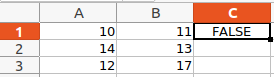To quickly propagate the formula across all desired cells in the column, select cell C1 (which now contains the formula) so that a bounding box appears around the cell.

Hover the mouse over the fill handle (small square at the bottom right corner of the bounding box) so that the mouse pointer turns into a crosshair, then click and drag the crosshair down the length of the column.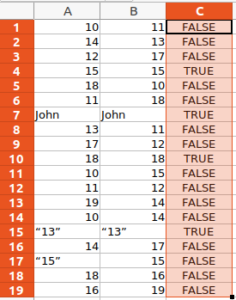Excel will populate the selected cells in column C, by copying the formula contained in C1 and automatically adjusting any cell references, thus producing the corresponding TRUE or FALSE output, which is dependent on the cell values from columns A and B for each row.

The results show that comparisons can not only be made between numeric values, but text as well.

The contents of cells A15 and B15 are considered as text, since they contain non-numeric characters (the double quotes).

The contents of the two cells are also deemed to be equal.

Cells A17 and B17 on the other hand, while they both contain the number 15, are not equal, since cell A17 is considered as text, and cell B17 as numeric.

## Boolean Type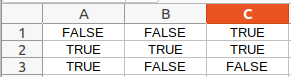While our data so far has contained numbers and text, cells can also contain boolean values (i.e. TRUE or FALSE).

The above shows comparisons for equality between cells containing boolean values and their respective result.

While it will almost never occur that someone would store boolean values directly in a spreadsheet, it is still good to have a basic understanding of the boolean type, since it plays a vital role when evaluating expressions.

Many Excel functions return a boolean result, as we will see in a while.

It is therefore important in a comparison, that in order for two cells to be considered as equal, that the contents of both cells should also be of the same type (i.e. text, numeric, boolean).

This also holds true for the other comparison operators, where cell content type can affect the outcome.

As a quick visual indicator, by default, within a cell, Excel left aligns text, centers boolean, and right aligns numeric values.

## White Space

One final and key point to keep in mind is white space.

White space most commonly includes the space character, line break (or enter), and tab.

White space does not print a visible character so is not always discernible, but it does affect the comparison.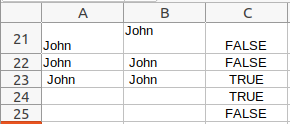Consider the above.

While adjacent cells in columns A and B may appear to have more or less the same content, they differ due to white space.

Cells A24 and B24 are both blank, showing that comparison using blank cells is also valid.

Although not visible, cell A25 is blank and cell B25 contains a space character, which therefore makes them different and produces a FALSE result.

Visually however, they, along with cells A24 and B24 all look identical.

Since white space can be a problem, if it is required that leading and trailing white space, as well as white space inside text should not adversely affect a comparison, Excel provides a couple of handy functions, TRIM and CLEAR, that can help take care of this.

TRIM removes leading and trailing spaces (but only spaces), as well as converting any multiple spaces between printing characters and words, to a single space character.

CLEAR removes all non-printing characters (i.e. the first 32 characters of the ASCII character set), such as new lines, carriage returns, and tabs.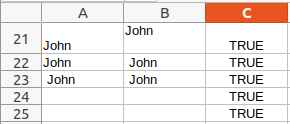The formula now becomes:

=TRIM(CLEAN(A21))=TRIM(CLEAN(B21))

The formula will produce the above results.

With all white space being filtered out by the two functions, all comparisons between adjacent cells in columns A and B now evaluate to TRUE.

Before moving on, while our comparisons so far have been about whether two cells have equal values or not, any of the comparison operators can be substituted in the formulas in place of the equals sign, to perform the desired comparison.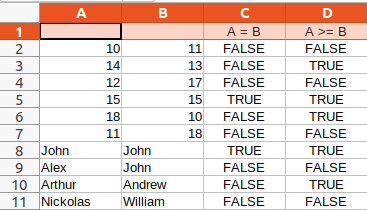As an example, the updated sheet shows the results of comparing the values, for both numeric and text values, in columns A and B, for equality (column C), and greater than or equal to (column D).

The formula for comparing for greater than or equal to is:

=A>=B

## The IF Function

Another way of writing the comparison formulas involves the use of Excel’s IF function.

Taking our original formula, =A1=B1, and using the IF function in its simplest form, our formula now becomes:

=IF(A1=B1).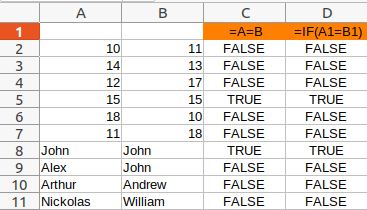As can be seen, the results are exactly the same, regardless of which formula is used.

So what is the difference or benefit in using the IF function?

Remember earlier when it was stated that the result of the comparison could be programmed to output something more meaningful than just the boolean TRUE or FALSE.

The IF function can also take additional parameters that define what is displayed, dependent on the result of the comparison.

For example, the formula =IF(A1=B1,”Yes”,“No”) will display Yes if the comparison evaluates to TRUE, and No if it evaluates to FALSE.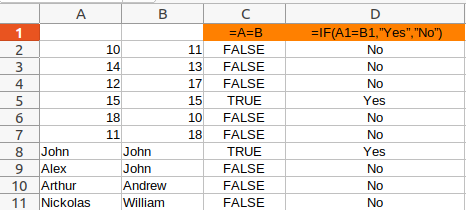The results in column D are now a little more meaningful.

And of course, the comparison could just as easily have been if one value is less than, greater than, or any of the other comparison operations.

But this is not all.

The IF function has a close relative, IFS, with some even more powerful features.

Consider the following which covers a variety of comparisons inside the one function.

It tests if the two values are equal, if one is greater than the other, or if it is less than the other, and outputs the corresponding text.

=IFS(A2=B2, ”Equal”, A2 >B2, ”Greater”, A2<B2, ”Less”)Now the formula displays one of Equal, Greater, or Less, depending on the result from the comparison between two cells.

While this is a simple example, the ability to display custom output, make IF and IFS very handy and powerful functions.

## Conditional Formatting

So far, we’ve compared two cells and displayed the result of the comparison in a third cell.

This time, we’ll see yet another way to highlight equality or differences when doing a comparison, with the aid of conditional formatting.

With conditional formatting, a formula is still used, but the result does not generate display in a third cell or column, but instead, the cells themselves are altered visually in some way, depending on the result.

This can be as simple as changing the cells font styles to bold or italics, or more commonly, the font color and cell background.

Excel’s conditional formatting is rules based, and several rules can be applied to the same set of cells.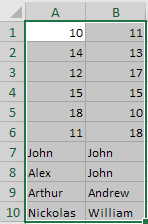Start by selecting all data cells in the comparison (do not include any headers if they exist).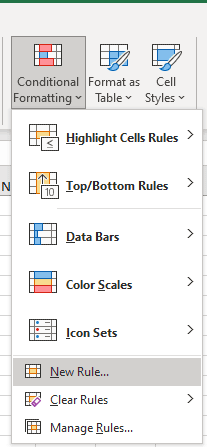Then, from Excel’s main navigation menu, open Home > Conditional Formatting > New Rule…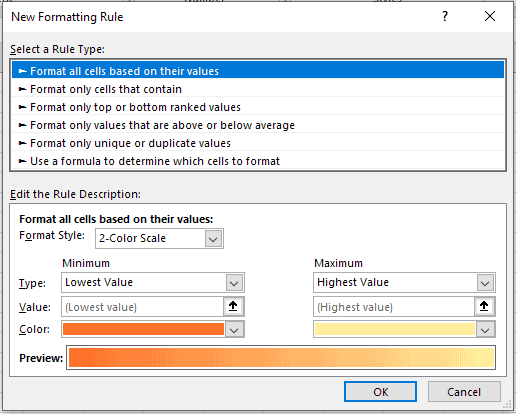This will bring up the New Formatting Rule dialog box.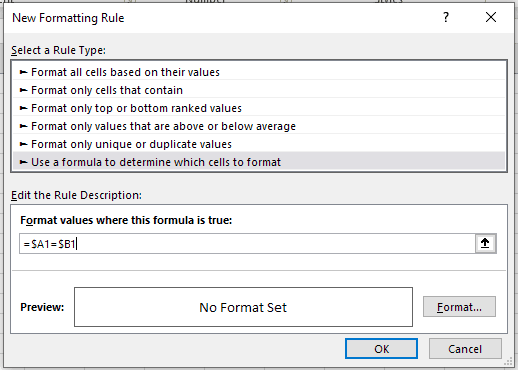From the top pane’s Select a Rule Type list, choose the option Use a formula to determine which cells to format.

In the lower pane, enter the formula =\$A1=\$B1 in the Format values where this formula is true input field.

The formula here is of the same format and uses the same operators as those we’ve been using in the formulas so far.

In this example, we are again testing for equality, but there is nothing to stop us from using any of the other comparisons.

Note that this time, our references to the cells include a preceding dollar sign (i.e. \$A1 and \$B1).

This is known as absolute referencing and is required in this case, otherwise the formatting will not affect both columns in our sheet.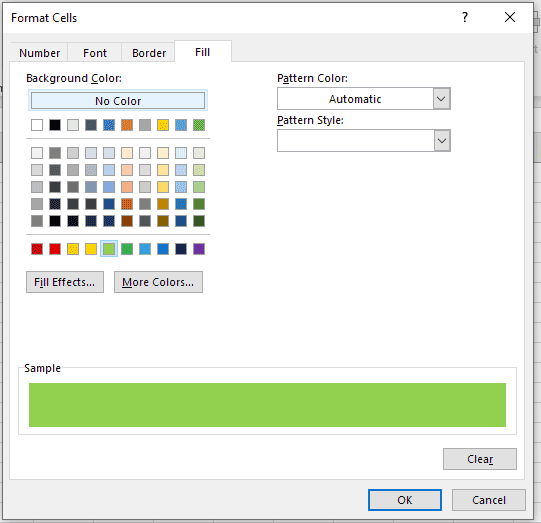Once the formula has been entered, click the Format… button to bring up the Format Cells dialog box.

For our purposes, we are going to use a background fill color, however we could just as easily have chosen to make the text bold, italics, strikethrough (from the Font tab).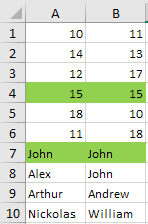After selecting a background fill color, click the OK button to return to the Edit Formatting Rule dialog box.

Clicking the OK button again, will apply the rule to the selected cells and the selected background color will now fill any cells that equate to TRUE for the entered formula.

It is possible to create several rules that apply to the same set of cells, and to have those cells filled with a different background color depending on which rule matches.

## Lookup

All of the above have focused on a one to one comparison between adjacent cells.

The result of the comparison in one row, is independent of the contents of the other rows.

But how do we go about doing a check of a random list of country names to see which, if any, belong to a particular group of countries or a continent?

This is no longer a one to one comparison, but instead requires a one to many lookup.

We can start by filling one column up with all countries belonging to the desired group or continent, and putting the random list of candidate countries in a second column.

The comparison would then not be between adjacent cells, but between each individual cell in the candidate country column, against all cells in the all countries column.

A match would occur when the contents of a candidate country match the contents of any cell in the all countries column.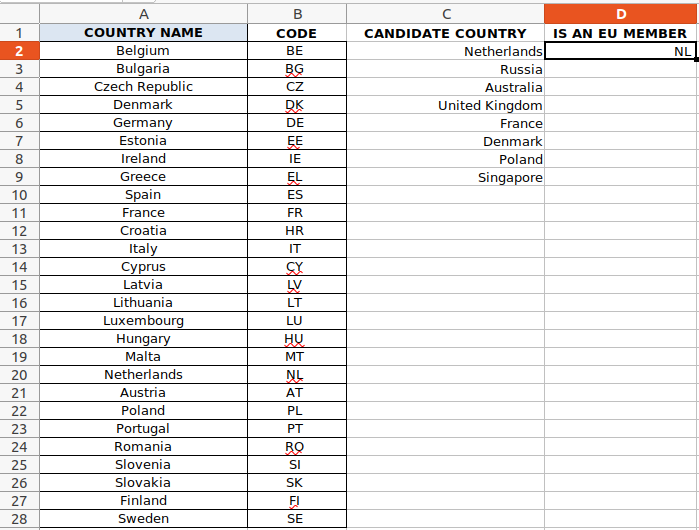The above sheet shows a list of European Union member countries in column A, and a random list of countries in column C.

We can no longer rely on the IF function since it is limited to comparing only a pair of cells.

Instead, we need to be able to do a lookup across a range of cells. VLOOKUP is an Excel function that does exactly this, namely to take a single cell’s value and do a lookup within a range of cell values, looking for a match.

Applying the formula:

=VLOOKUP(C2, \$A\$2:\$B\$28, 2, FALSE) to cell D2 results in a match and prints the country’s 2 letter code, NL.

Notice that the formula not only searches for a match, but if one is found, it also pulls the resulting output from column B (CODE), and displays it in column D.

VLOOKUP takes four parameters:

• The cell reference containing the value to lookup – in this case C2, which means we are requesting a lookup for Netherlands.
• The range of values to look through – A2 to B28. Column A has the full list of E.U. member country names and column B the corresponding country code. We are including two columns in the range, even though only the first column of the two (COUNTRY NAME), will be used in the comparison, because the resulting output is being pulled from the second column (CODE).
• Since the range of cells to look through covers two columns (A2 to B28), the column number in the formula, namely 2, specifies if a match is found, then return the result from the 2nd of the two columns in the range (i.e. column B, CODE).
• Whether the comparison should check for an exact match (FALSE) or approximate match (TRUE). This parameter is optional and defaults to TRUE if not provided.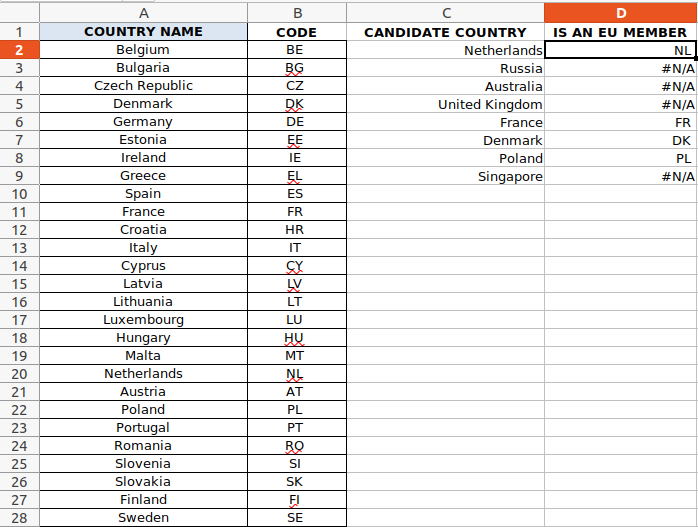If we now extend the formula in cell D2 to cover the remaining countries in the CANDIDATE COUNTRY column (by selecting cell D2, grabbing the fill handle in the bounding box and dragging it down to cell D9), we end up with the above results.

For Netherlands, France, Denmark and Poland, the corresponding 2 letter code has been returned, confirming there was a match.

For the remaining countries however, there was no match, but the resulting output is an ugly looking #N/A.

To fix this, we can wrap the formula in an IF function and test for whether the result from VLOOKUP is NA with another function, ISNA.

The formula now becomes:

=IF(ISNA(VLOOKUP(C2, \$A\$2:\$B\$28, 2, FALSE)) = TRUE, “-“, VLOOKUP(C2, \$A\$2:\$B\$28, 2, FALSE))

If the result of any lookup is #N/A, it is replaced by a hyphen, otherwise the country’s 2 letter code is printed.

This is now much cleaner and easier to read.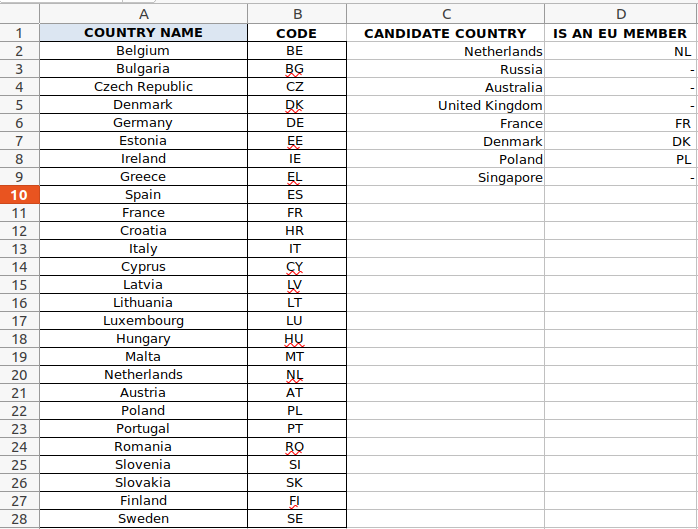While the above is a great improvement over the first VLOOKUP formula, the formula itself is a bit long-winded and messy.

Excel has an improved lookup function, XLOOKUP, that makes life even easier.

=XLOOKUP(C9, \$A\$2:\$A\$28, \$B\$2:\$B\$28,”-“, FALSE, TRUE)

XLOOKUP takes up to 6 parameters.

• Lookup value – The value to search for
• Lookup array – The range of values to search through for a match
• Return array – The range of values from which to return a result if a match is found
• If not found – if a valid match is not found, then instead of the default #N/A, the text defined here is returned. This parameter is optional.
• Match mode – (optional)
• 0 – Exact match. If none found, return #N/A. This is the default.
• -1 – Exact match. If none found, return the next smaller item.
• 1 – Exact match. If none found, return the next larger item.
• 2 – A wildcard match where *, ?, and ~ have special meaning.
• Search mode – Specify the search mode to use (optional)
• 1 – Perform a search starting at the first item. This is the default.
• -1 – Perform a reverse search starting at the last item.
• 2 – Perform a binary search that relies on lookup array being sorted in ascending order. If not sorted, invalid results will be returned.
• -2 – Perform a binary search that relies on lookup array being sorted in descending order. If not sorted, invalid results will be returned.

## Comparing Two Columns

As a final example, we’ll examine another case of comparing two columns.

This time we want to know if any value in any of the cells in one column is equal to, less than, or greater than any value in any of the cells of a second column.For example, we have two columns with the average monthly daytime temperatures in degrees Fahrenheit.

Column D for what we’ve called the winter months, November to April inclusive, and column B for the summer months, May to October inclusive.

We want to confirm that all the summer temperatures are above all of the winter temperatures, without being interested in individual months.

We just want a confirmation that the condition holds true.

We can do this using either the OR or AND function.

The following formula:

=OR(B2:B7<D2:D7)

Performs a logical OR of all the results returned from comparing all values in the cells B2 to B7 being less than all values in the cells D2 to D7.

The definition of the OR function is as follows:

The OR function returns TRUE if any of its arguments evaluate to TRUE, and returns FALSE if all of its arguments evaluate to FALSE.

According to this definition, if any temperature in the summer months was lower than any temperature in the winter months, the comparison would return TRUE, and so would our formula.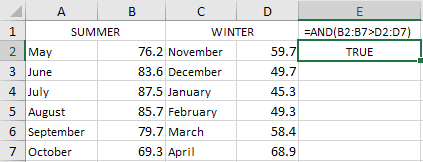Another way of approaching this is to confirm that all summer temperatures are above all the winter temperatures, with the formula:

=AND(B2:B7>D2:D7).

The definition for the AND function is:

The AND function returns TRUE if all its arguments evaluate to TRUE, and returns FALSE if one or more arguments evaluate to FALSE.

In other words, as long as all the summer temperatures remain higher than all the winter temperatures, the result will be TRUE.

## Conclusion

Comparing data between cells, or two (or more) columns, is very common in spreadsheets.

While we’ve used very simple formulas in our examples, more complex ones can be written to help detect fine or coarse, great or small deviations in almost any kind of data.

Such comparisons are possible thanks to Excel’s comparison operators, where two values can be checked to see if they are equal, or to determine which of the two is greater or smaller.

The result can be displayed as simple text output, or for something more elaborate, matching rows can be color highlighted.

However, not only can adjacent cells be compared, but a lookup can also be carried out comparing the value of one cell against a range of values in a column of cells.

While this article has been written with reference to Excel, with the exception of the XLOOKUP function, which is unique to Excel, all examples with minor differences, also apply to Google sheets and other spreadsheet applications, like LibreOffice Calc.

Although there’s no direct replacement for XLOOKUP, Google sheets has the equivalent and possibly more powerful, FILTER function.

And there’s always the traditional INDEX(MATCH) combination that work with all spreadsheets.

Costas has a wide range of experience in Information Technology covering computer hardware, programming, telecommunications, networking, web services, and general IT support. He's worked in various roles such as PHP programmer and web developer, technical and desktop support, hardware repair, system administration. Costas has excellent background in Microsoft Windows and Office Suite (Excel, Word, Powerpoint, etc), as well as a thorough understanding of Networking and Hardware maintenance.